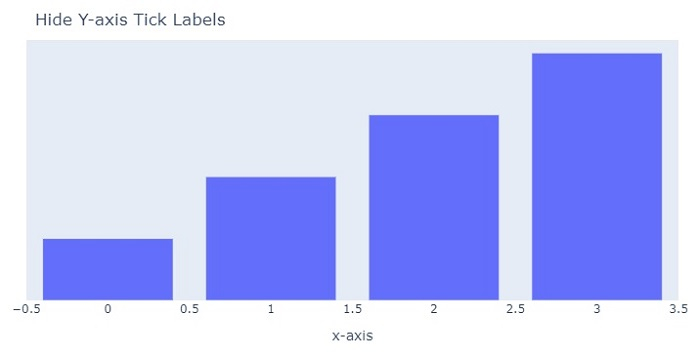# How to hide the Y-axis tick labels on a chart in Python Plotly?

Plotly is an open-source Python plotting library for creating charts. Python users can use Plotly to create interactive web-based visualizations. It can also be used in static document publishing and desktop editors such as PyCharm and Spyder.

In this tutorial, we will show how you can hide the Y-axis tick labels on a Plotly chart −

• Here, we will use the plotly.graph_objects module to generate figures. It contains a lot of method to generate charts.

• In addition, we will use the Layout method and its properties, showticklabels and visible" to show/hide the tick labels.

Follow the steps given below the hide the Y-axis tick labels on a chart.

Step 1

Import the plotly.graphs_objs module and alias as go.

import plotly.graphs_objs as go


Step 2

Create a bar chart to set the layout and title values.

fig = go.Figure(
data=[go.Bar(y=[10,20,30,40])],
layout_title_text="Hide y-axis",
layout = {
'xaxis': {'title': 'x-axis',
'visible': True,
'showticklabels': True},
'yaxis': {'title': 'y-axis',
'visible': False,
'showticklabels': False},
},
)


Step 3

Set the margin coordinates for all the direction.

'margin': dict(
l = 20, # left
r = 15, # right
t = 30, # top
b = 40, # bottom
),


## Example

The complete code to hide Y-axis label as follows,

import plotly.graph_objects as go
fig = go.Figure(
data=[go.Bar(y=[10,20,30,40])],
layout_title_text="Hide Y-axis Tick Labels",
layout = {
'xaxis': {'title': 'x-axis', 'visible': True, 'showticklabels': True},

'yaxis': {'title': 'y-axis', 'visible': False, 'showticklabels': False},

# specify margins in px
'margin': dict(
l = 20, # left
r = 15, # right
t = 30, # top
b = 40, # bottom
),
},
)
fig.update_layout(width=716, height=350)
fig.show()


## Output

On execution, it will show the following output on the browser −Observe that the Y-axis tick labels are hidden in the chart.